library(ggplot2) # for general plotting
library(car)     # for ANOVA (Type II used, better than Type I when there is an unbalanced design)

## Information of data source

Damage from TU and TU-A (100 mites) on Moneymaker tomato leaflets, 24 hpi.

## Read in the data and view structure to identify any issues in data formatting

damage.data <- read.csv("~/Lab Stuff/Adapted mites/Tomato/Damage assay/TU and TA on Moneymaker/Damage R data.csv", header = TRUE)

# trial as a factor
damage.data$Trial <- factor(damage.data$Trial)

str(damage.data)
## 'data.frame':    24 obs. of  3 variables:
##  $Trial : Factor w/ 3 levels "1","2","3": 1 1 1 1 2 2 2 2 3 3 ... ##$ Mite.Strain: Factor w/ 2 levels "TU","TU-A": 1 1 1 1 1 1 1 1 1 1 ...
##  $Damage : num 0.312 0.125 0.188 0.125 0.375 ... ## Formulate hypothesis H0: There will be no difference in damage produced by the mite strains. HA: TU-A will produce more damage on Moneymaker leaflets than TU mites. ## Conduct data exploration ### Outliers in the response variable (Damage) within explanatory variables (Trial, Mite.Strain). ggplot(damage.data, aes(x = Trial, y = Damage)) + geom_boxplot() + theme_classic()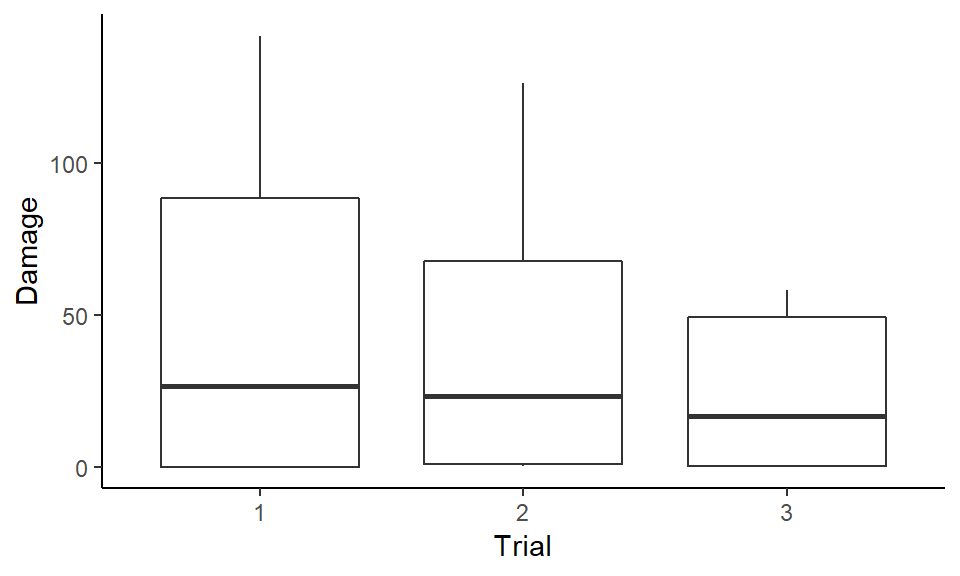ggplot(damage.data, aes(x = Mite.Strain, y = Damage)) + geom_boxplot() + theme_classic()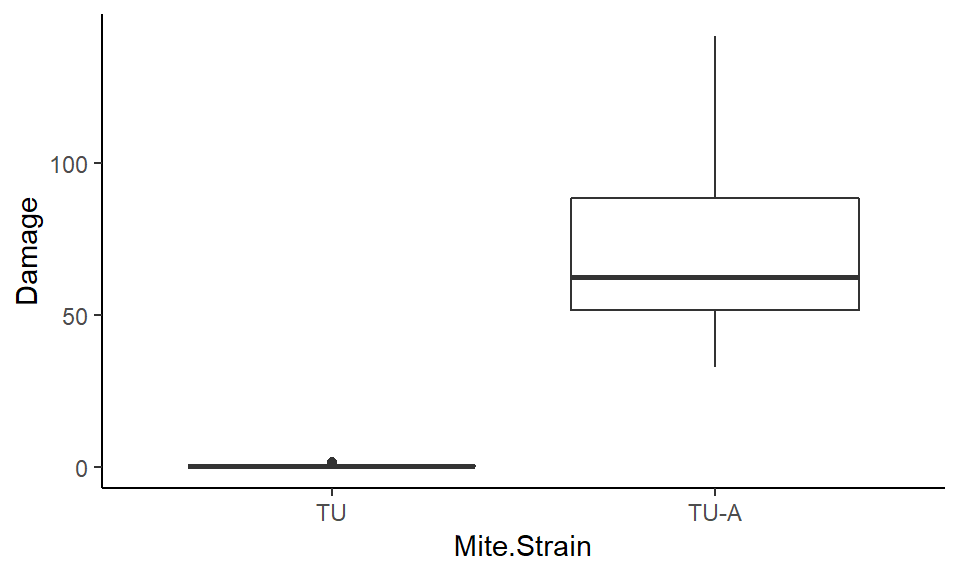Outlier left in, probably represents real variability. ### Collinearity of the explanatory variables Des not apply, all explanatory variables are categorical/factorial. ### Spatial/temporal or other hierarchical aspects of sampling design No, I am treating Trial as a main effect to check for reproducibility (not a random effect/blocking factor). ### Interactions (is the quality of the data good enough to include them?) Interaction betweenTrial and Mite.Strain will be performed to test for reproducibility. ### Zero inflation in Y No ### Are categorical covariates balanced? summary(damage.data) ## Trial Mite.Strain Damage ## 1:8 TU :12 Min. : 0.125 ## 2:8 TU-A:12 1st Qu.: 0.375 ## 3:8 Median : 17.312 ## Mean : 36.885 ## 3rd Qu.: 60.469 ## Max. :142.000 Yes ## Apply model # fit linear model and display model fit information and ANOVA table m <- lm(Damage ~ Mite.Strain + Trial + Mite.Strain:Trial, data = damage.data) summary(m) ## ## Call: ## lm(formula = Damage ~ Mite.Strain + Trial + Mite.Strain:Trial, ## data = damage.data) ## ## Residuals: ## Min 1Q Median 3Q Max ## -40.484 -3.188 -0.063 0.168 48.922 ## ## Coefficients: ## Estimate Std. Error t value Pr(>|t|) ## (Intercept) 0.1875 10.5359 0.018 0.9860 ## Mite.StrainTU-A 93.2969 14.9000 6.262 6.63e-06 *** ## Trial2 0.8281 14.9000 0.056 0.9563 ## Trial3 0.2500 14.9000 0.017 0.9868 ## Mite.StrainTU-A:Trial2 -16.7969 21.0717 -0.797 0.4358 ## Mite.StrainTU-A:Trial3 -45.0625 21.0717 -2.139 0.0464 * ## --- ## Signif. codes: 0 '***' 0.001 '**' 0.01 '*' 0.05 '.' 0.1 ' ' 1 ## ## Residual standard error: 21.07 on 18 degrees of freedom ## Multiple R-squared: 0.8176, Adjusted R-squared: 0.7669 ## F-statistic: 16.13 on 5 and 18 DF, p-value: 4.217e-06 Anova(m) ## Anova Table (Type II tests) ## ## Response: Damage ## Sum Sq Df F value Pr(>F) ## Mite.Strain 31692 1 71.3750 1.118e-07 *** ## Trial 2054 2 2.3127 0.1277 ## Mite.Strain:Trial 2074 2 2.3360 0.1253 ## Residuals 7992 18 ## --- ## Signif. codes: 0 '***' 0.001 '**' 0.01 '*' 0.05 '.' 0.1 ' ' 1 # Calculate effect size and display result.anova<-Anova(m) ss<-result.anova$"Sum Sq"    ##ss = sum of squares
pes<-ss/(ss+ss[length(ss)])  ##pes = partial e squared
pes[length(pes)]<-""
result.anova$"Part E Sq"<-pes result.anova ## Anova Table (Type II tests) ## ## Response: Damage ## Sum Sq Df F value Pr(>F) Part E Sq ## Mite.Strain 31692 1 71.3750 0.00000 0.79860 ## Trial 2054 2 2.3127 0.12766 0.20444 ## Mite.Strain:Trial 2074 2 2.3360 0.12532 0.20607 ## Residuals 7992 18 # plot interactions interaction.plot(damage.data$Mite.Strain, damage.data$Trial, damage.data$Damage, type="l", leg.bty="o", leg.bg="grey95", lwd=2, ylab="Damage", xlab="Mite Strain", main="Mite.Strain:Trial")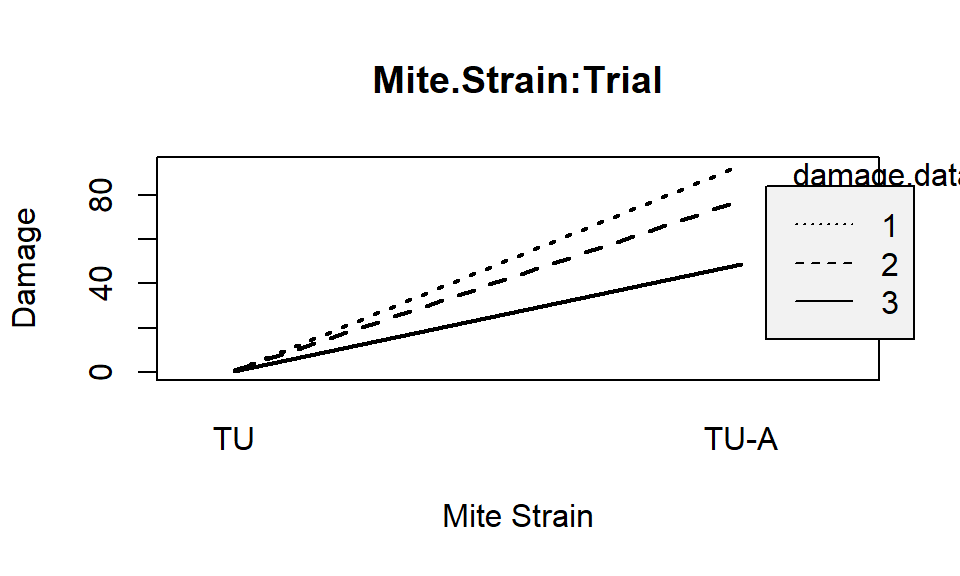## Validate model

damage.data$m.fit <- fitted(m) # fitted values damage.data$m.res <- rstandard(m) # Pearson residuals

### Residual distribution / Overdispersion

We assumed normal residuals. This is the least important regression assumption but its can be tested with a qq plot.

ggplot(damage.data, aes(sample = m.res)) + geom_qq() +
geom_abline(intercept = 0, slope = 1) + theme_classic()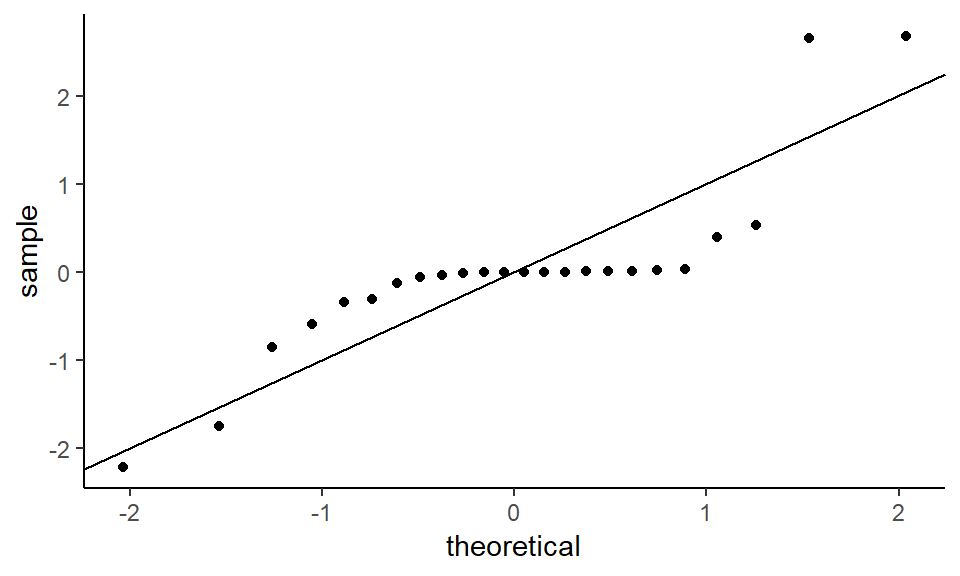Some curvage here, not too bad.

### Residuals vs fitted values

Testing for:

Linearity - there should be no curvilinear pattern in the residuals.

Equal variance - the vertical spread of the residuals should be constant across all fitted values.

ggplot(damage.data, aes(x = m.fit, y = m.res)) +
geom_point() + geom_hline(yintercept = 0) + geom_smooth() + theme_classic()
## geom_smooth() using method = 'loess' and formula 'y ~ x'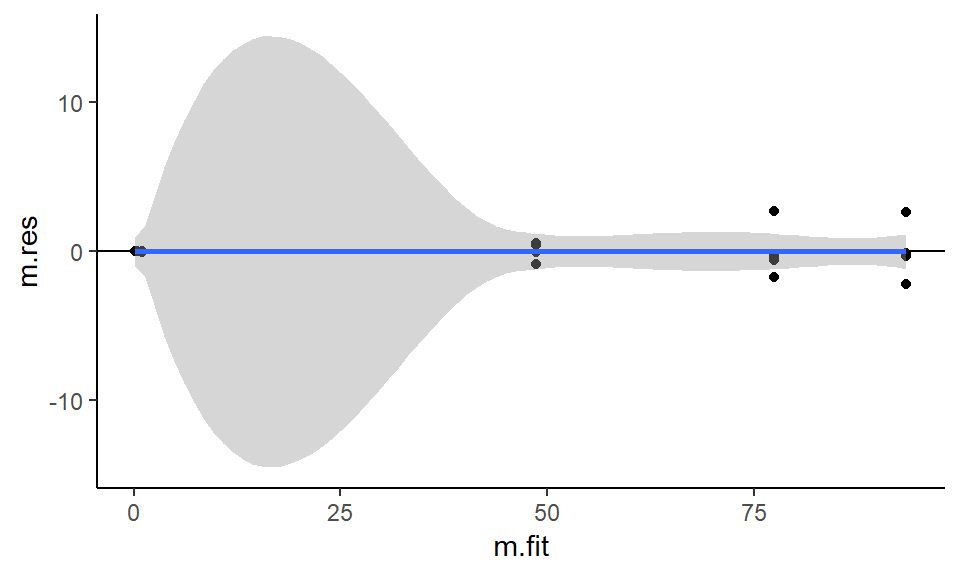Linearity - decent - confidence interval includes 0 for all values.

Equal variance - no obvious problems

### Residuals vs explanatory variables

Should be centered around 0, if not then model requires another explanatory variable(s), to account for observed variation.

ggplot(damage.data, aes(x = Mite.Strain, y = m.res)) +
geom_boxplot() + geom_hline(yintercept = 0) + theme_classic()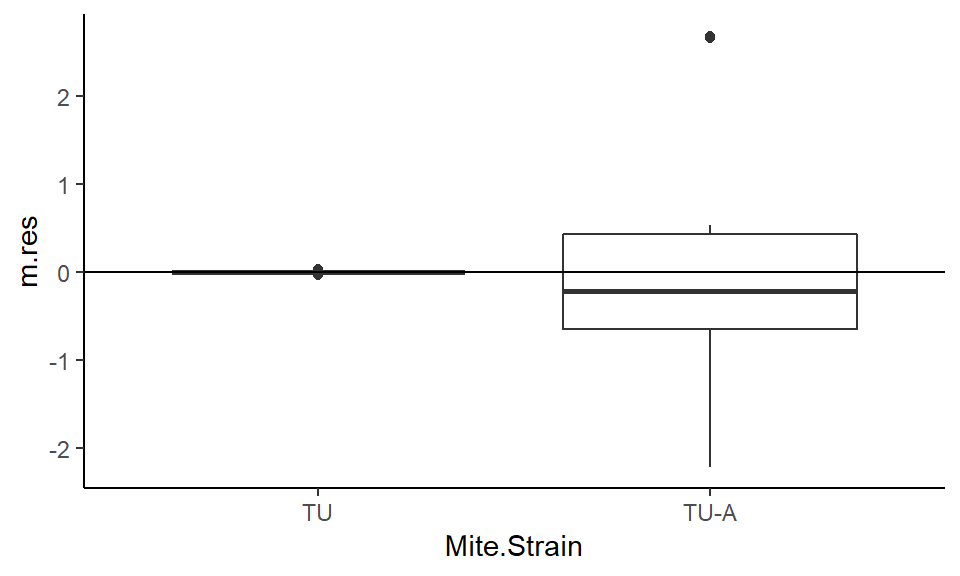ggplot(damage.data, aes(x = Trial, y = m.res)) +
geom_boxplot() + geom_hline(yintercept = 0) + theme_classic()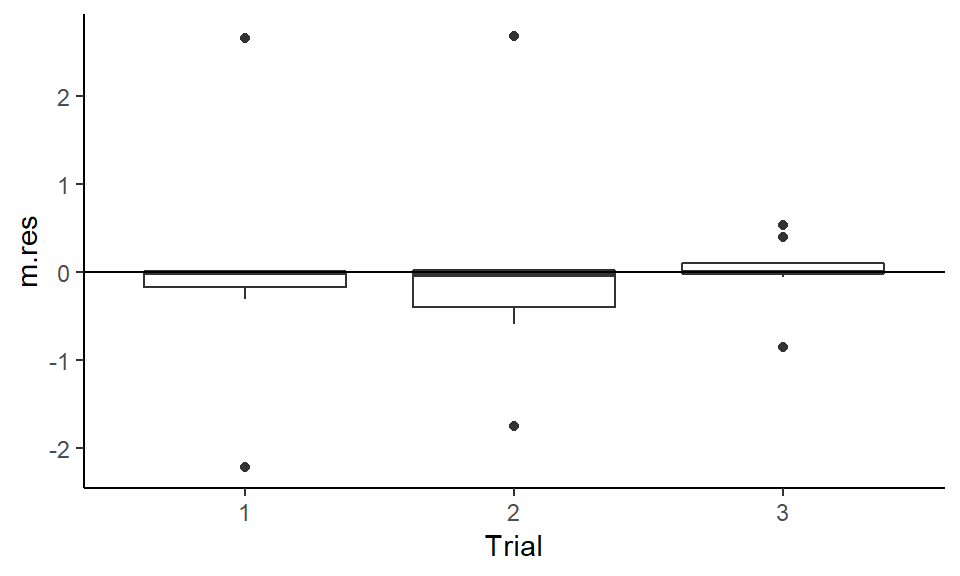ggplot(damage.data, aes(x = Mite.Strain:Trial, y = m.res)) +
geom_boxplot() + geom_hline(yintercept = 0) + theme_classic()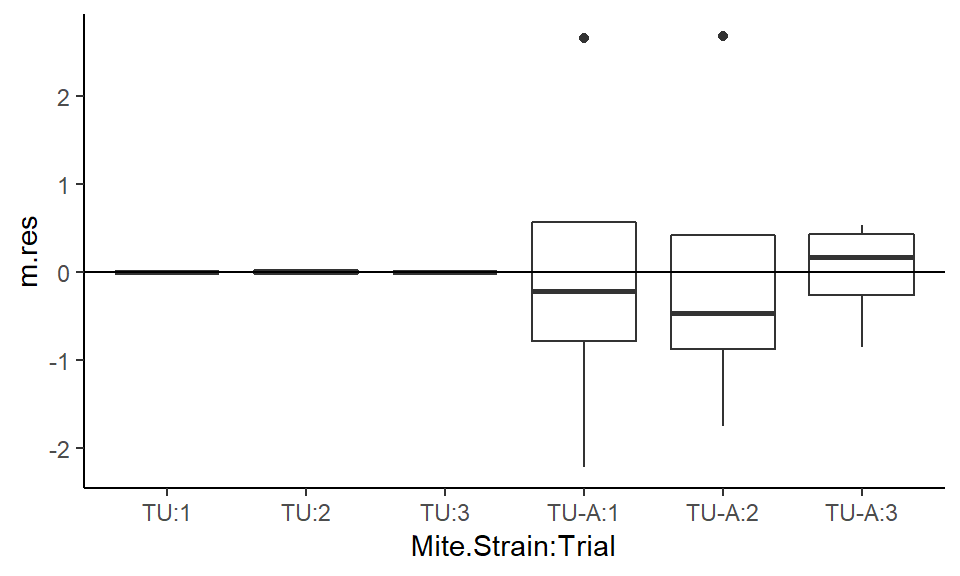Looks good.

Model is valid and interpretation of ANOVA is good.

sessionInfo()
## R version 3.6.0 (2019-04-26)
## Platform: x86_64-w64-mingw32/x64 (64-bit)
## Running under: Windows 10 x64 (build 17763)
##
## Matrix products: default
##
## locale:
##
## attached base packages:
##  stats     graphics  grDevices utils     datasets  methods   base
##
## other attached packages:
##  car_3.0-3     carData_3.0-2 ggplot2_3.1.1
##
## loaded via a namespace (and not attached):
##   zip_2.0.2         Rcpp_1.0.1        cellranger_1.1.0
##   pillar_1.4.1      compiler_3.6.0    plyr_1.8.4
##   forcats_0.4.0     tools_3.6.0       digest_0.6.19
##  evaluate_0.14     tibble_2.1.1      gtable_0.3.0
##  pkgconfig_2.0.2   rlang_0.3.4       openxlsx_4.1.0
##  curl_3.3          yaml_2.2.0        haven_2.1.0
##  xfun_0.7          rio_0.5.16        withr_2.1.2
##  stringr_1.4.0     knitr_1.23        hms_0.4.2
##  lazyeval_0.2.2    munsell_0.5.0     crayon_1.3.4